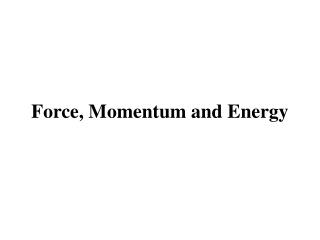# Force, Momentum and Energy - PowerPoint PPT PresentationDownload PresentationForce, Momentum and Energy

Force, Momentum and EnergyDownload Presentation## Force, Momentum and Energy

- - - - - - - - - - - - - - - - - - - - - - - - - - - E N D - - - - - - - - - - - - - - - - - - - - - - - - - - -
##### Presentation Transcript

1. Force, Momentum and Energy

2. Newton’s Laws of Motion • Our understanding of how an object reacts to force, or how the motion of an object is affected by force, is summarized by Newton’s Laws of Motion: • First Law of Motion • In the absence of a net force, an object moves with constant velocity. • Second Law of Motion • Force = mass  acceleration, • or, • F = m a • Third Law of Motion • For any force, there is always an equal and opposite reaction force.

3. First Law of Motion In the absence of a net force, an object moves with constant velocity • An object at rest will remains at rest unless a net force is applied to it. • An object in motion will continue to move with the same speed in the same direction unless a net force is applied to it. • Examples: • Spacecraft does not need to have its rocket engine on all the time to keep moving in space. • Air hockey!

4. Second Law of Motion F = m a, or, Eamples: • Heavy trucks (large m) accelerate very slowly… • Sport cars accelerate real fast (0-60 mph in 5 seconds?). • Throwing base balls.

5. Third Law of Motion For every force, there is always an equal and opposite reaction force. Eamples: • The rocket launch. • When you hit somebody with your hand, your hand hurts also.

6. Newton’s Law of Gravity • Every mass attracts every other mass through the force of gravity. • The strength of the gravitational force is directly proportional to the product of their masses. • The strength of the gravitational force between two objects decreases with the square of the distance between them. This is the inverse square law. • The Gravitational Constant G = 6.67 × 10-11 m3/(kg × s2)

7. Does rock falls faster than feather? Click image to start movie On the surface of the Earth, the gravitational acceleration experienced by all the objects is It does not depends on the mass of the object! Does heavier object falls faster than lighter object? • All the objects falling off the roof would reach the ground at the same time, independent of their masses (click to start movie)